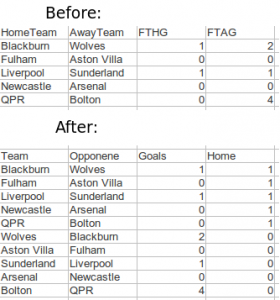# Predicting football results with Poisson regression pt. 1

I have been meaning to write about my take on using Poisson regression to predict football results for a while, so here we go. Poisson regression is one of the earliest statistical methods used for predicting football results. The goal here is to use available data to to say something about how many goals a team is expected to score and from that calculate the probabilities for different match outcomes.

The Poisson distribution
The Poisson distribution is a probability distribution that can be used to model data that can be counted (i.e something that can happen 0, 1, 2, 3, … times). If we know the number of times something is expected to happen, we can find the probabilities that it happens any number of times. For example if we know something is expected to happen 4 times, we can calculate the probabilities that it happens 0, 1, 2, … times.

It turns out that the number of goals a team scores in a football match are approximately Poisson distributed. This means we have a method of assigning probabilities to the number of goals in a match and from this we can find probabilities for different match results. Note that I write that goals are approximately Poisson. The Poisson distribution does not always perfectly describe the number of goals in a match. It sometimes over or under estimates the number of goals, and some football leagues seems fit the Poisson distribution better than others. Anyway, the Poisson distribution seems to be an OK approximation.

The regression model
To be able to find the probabilities for different number of goals we need to find the expected number of goals L (It is customary to denote the expectation in a Poisson distribution by the Greek letter lambda, but WordPress seem to have problems with greek letters so i call i L instead). This is where the regression method comes in. With regression we can estimate lambda conditioned on certain variables. The most obvious variable to look at is which team is playing. Manchester United obviously makes more goals than Wigan. The second thing we want to take into account is who the opponent is. Some teams are expected to concede fewer goals, while others are expected to let in more goals. The third thing we want to take into account is home field advantage.

Written in the language of regression models this becomes

log(L) = mu + home + teami + opponentj

The mu is the overall mean number of goals. The home is the effect on number of goals a team has by playing at home. Teami is the effect of team number i, opponentj is the effect of team j.

(Note: Some descriptions of the Poisson regression model on football data uses the terms offensive and defensive strength to describe what I have called team and opponent. The reason I prefer the terms I use here is because it makes it a bit easier to understand later when we look at the data set.)

The logarithm on the left hand side is called the link function. I will not dwell much on what a link function is, but the short story is that they ensure that the parameter we try to estimate don’t fall outside its domain. In this case it ensures us that we never get negative expected number of goals.

Data
In my example I will use data from football-data.co.uk. What data you would want to use is up to yourself. Typically you could choose to use data from the last year or the least season, but that is totally up to you to decide.

Each of the terms on the right hand side of the equation (except for mu) corresponds to a columns in a table, so we need to fix our data a bit before we proceed with fitting the model. Each match is essentially two observations, one for how many goals the home team scores, the second how many the away team scores. Basically, each match need two rows in our data set, not just one.

Doing the fix is an easy thing to do in excel or Libre Office Calc. We take the data rows (i.e. the matches) we want to use and duplicate them. Then we need to switch the away team and away goals columns so they become the same as the home team column. We also need a column to indicate the home team. Here is an example on how it will look like:In the next part I will fit the actual model, calculate probabilities and describe how we can make predictions using R.